Home  - Pure_And_Applied_Math - Calculus
e99.com Bookstore
 Images Newsgroups
 61-80 of 176    Back | 1  | 2  | 3  | 4  | 5  | 6  | 7  | 8  | 9  | Next 20

Calculus:     more books (100)
1. Precalculus: Mathematics for Calculus, Enhanced Review Edition (with CD-ROM and iLrn? Printed Access Card) by James Stewart, Lothar Redlin, et all 2007-02-05
2. Quick Calculus: A Self-Teaching Guide, 2nd Edition by Daniel Kleppner, Norman Ramsey, 1985-10-28
3. Essential Calculus: Early Transcendentals by James Stewart, 2006-03-01
4. Calculus by Ron Larson, 2000-11
5. Homework Helpers: Pre-Calculus by Denise, Ph.D. Szecsei, 2007-05-30
6. Study Guide for Stewart's Single Variable Calculus, 6th by Richard St. Andre, 2007-05-23
7. Single Variable Calculus: Early Transcendentals Student Solutions Manual by Jon Rogawski, 2007-08-09
8. Student Solutions Manual for Stewart/Redlin/Watson's Precalculus: Mathematics for Calculus, 5th by James Stewart, Lothar Redlin, et all 2005-10-07
9. Calculus: Concepts and Contexts (Stewart's Calculus Series) by James Stewart, 2009-03-09
10. Barron's AP Calculus with CD-ROM by Shirley O. Hockett, David Bock, 2010-02-01
11. Calculus: Early Transcendental Functions by Ron Larson, Robert P. Hostetler, et all 2006-01-03
12. Advanced Calculus by David Widder, 2009-01-23
13. Calculus With Analytic Geometry by George Simmons, 1996-10-01
14. The Calculus of Friendship: What a Teacher and a Student Learned about Life while Corresponding about Math by Steven Strogatz, 2009-08-03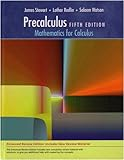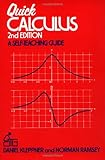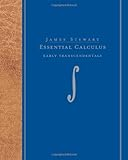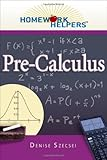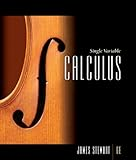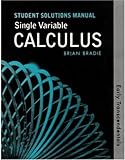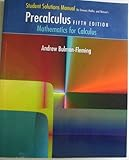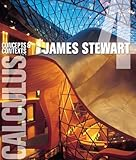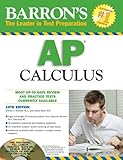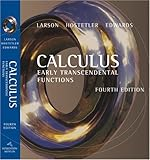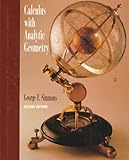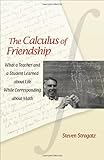lists with details

1. Non-Newtonian Calculus
Includes discussions of general theory and heuristic guides for application.
http://www.geocities.com/nonnewtoniancalculus/

Extractions: The non-Newtonian calculi were created in the period from 1967 to 1970 by Michael Grossman and Robert Katz. These calculi provide a wide variety of mathematical tools for use in science, engineering, and mathematics. They appear to have considerable potential for use as alternatives to the classical calculus of Newton and Leibniz. The first non-Newtonian calculus is the topic of Grossmans book "The First Nonlinear System of Differential and Integral Calculus" (QA303.G878). In that calculus the exponential functions play the role that the linear functions play in the classical calculus. A non-Newtonian calculus in which the power functions play that role is presented in Grossmans book "Bigeometric Calculus: A System with a Scale-Free Derivative (QA306.G84). Each non-Newtonian calculus, as well as the classical calculus, can be weighted in a manner explained in the book "The First Systems of Weighted Differential and Integral Calculus (QA303.G876) by Jane Grossman, Michael Grossman, and Robert Katz. Natural outgrowths of the systems of weighted calculus are the systems of meta-calculus, which are described in Jane Grossman's book "Meta-Calculus: Differential and Integral (QA303.G877).

2. Toolkit
The MathServ calculus Toolkit. During periods of heavy use, MathServ can be very slow to respond. Please do not resubmit jobs during
http://mss.math.vanderbilt.edu/~pscrooke/toolkit.html

3. Oresme, Nicole
Concise biography with a note on his mathematical contributions.
http://occawlonline.pearsoned.com/bookbind/pubbooks/thomas_awl/chapter1/medialib

Extractions: Oresme, Nicole (ca. 13201382) Frenchman Oresme went to the University of Paris in the 1340s, studying theology and liberal arts. Later he was a faculty member and administrator at the same university. Oresme served royalty as an educator and a scholar and, therefore, had support for his research. He translated Aristotle into French. Eventually he was made a bishop. He began to think about mathematics and in particular rates of change, like velocity and acceleration. His work entitled De configurationibus (1350s) contained results in geometry and was the first to present graphs of velocities. The argument we use to show the divergence of the harmonic series was devised by Oresme in this publication. Oresme was a popularizer of science and did not believe in Albert of Saxonys generally accepted model of free fall. Oresme preferred Aristotles constant-acceleration model, which became popular among Oxford scholars in the 1330s and was eventually refined and tested by Galileo three hundred years later. In another publication

4. THE CALCULUS PAGE PROBLEMS LIST
THE calculus PAGE PROBLEMS LIST. Problems and Solutions Developed by DA Kouba. And brought to you by ecalculus.org. Beginning Differential calculus
http://www.math.ucdavis.edu/~kouba/ProblemsList.html

Extractions: Problems and Solutions Developed by : And brought to you by : Problems on the continuity of a function of one variable Problems on the "Squeeze Principle" Problems on the limit definition of the derivative Problems on the chain rule Problems on the product rule Problems on the quotient rule Problems on differentiation of trigonometric functions Problems on differentiation of inverse trigonometric functions Problems on detailed graphing using first and second derivatives Problems on applied maxima and minima Problems on implicit differentiation Problems on related rates Problems on logarithmic differentiation Problems on the differential Problems on the Intermediate-Value Theorem Problems on the Mean Value Theorem Problems using summation notation Problems on the limit definition of a definite integral Problems on u-substitution Problems on integrating exponential functions Problems on integrating trigonometric functions Problems on integration by parts Problems on integrating certain rational functions, resulting in

5. ESAIM Control, Optimisation And Calculus Of Variations
Part of European Series in Applied and Industrial Mathematics. Full text from vol.1 (1995).
http://www.edpsciences.com/cocv/

6. THE CALCULUS PAGE
THE calculus PAGE HAS A NEW ADDRESS ! See http//ecalculus.Org.
http://www.math.ucdavis.edu/~kouba/CalculusPage.html

7. Focus On Calculus:
Topics in calculus that are important in physics
http://omega.albany.edu:8008/mat214dir/Baierlein.html

Extractions: What topics in calculus would physicists like to see their students learn? A lot of topics, of course, but the need to economize in this article forces me to economize in what I might ask of a calculus teacher, who is strapped for time, if not for space. Here are some highlights- some areas of especial concern to the study of physics. Exponentials and logarithms That the derivative of an exponential function is proportional to the function itself is the most important property of those functions. A physicist would like to start with that property, as displayed here: Numerical exploration with base (b=2) yields a coefficient of b X that is less than 1; trying (b = 10) yields a coefficient greater than 1. In between 2 and 10 there ought to be a number that yields 1, and thereby e enters the scene. Then it is a matter of small, relatively easy steps to develop the topic and to finish with ln y as the integral of one-over-x dx. That logarithmic relation always puzzles students, and so it is best to place it last, not at the start, where it might derail the entire development. Expansions and approximations Almost every ``exactly-solved'' problem in physics is based on some initial approximation. To be sure, solutions have become famous as exact solutions to nonlinear differential equations, but those equations themselves are merely approximations to more fundamental equations. Physics students need to become handy with the Taylor expansion and the binomial expansion. Their level of expertise should enable them to apply those expansions to functions like

8. Math Forum: Calculus
The best Internet resources for calculus classroom materials, software, Internet projects, and public forums for discussion. calculus. Back to Math by Subject
http://mathforum.org/calculus/calculus.html

9. Calculus History
The main ideas of calculus developed over a very long period of time. Read about some of the mathematicians who contributed to this field of mathematics.
http://www-groups.dcs.st-and.ac.uk/~history/HistTopics/The_rise_of_calculus.html

Extractions: The main ideas which underpin the calculus developed over a very long period of time indeed. The first steps were taken by Greek mathematicians. To the Greeks numbers were ratios of integers so the number line had "holes" in it. They got round this difficulty by using lengths, areas and volumes in addition to numbers for, to the Greeks, not all lengths were numbers. Zeno of Elea , about 450 BC, gave a number of problems which were based on the infinite. For example he argued that motion is impossible:- If a body moves from A to B then before it reaches B it passes through the mid-point, say B of AB. Now to move to B it must first reach the mid-point B of AB . Continue this argument to see that A must move through an infinite number of distances and so cannot move. Leucippus Democritus and Antiphon all made contributions to the Greek method of exhaustion which was put on a scientific basis by Eudoxus about 370 BC. The method of exhaustion is so called because

10. Calculus Bible
"The calculus Bible" by GS Gill.
http://www.math.byu.edu/Math/CalculusBible/

11. Math Forum: Algebra & Calculus Sketches - Ruth Carver
The Math Forum Corner for Interactive Geometry Software. Algebra and calculus Sketches. by Ruth Carver. calculus. Tangent Line Problem.
http://mathforum.org/sum95/ruth/sketches/algcalc.sketches.html

12. Mathematics Reference
Trigonometry identities and calculus rules for integration and differentiation.
http://www.alcyone.com/max/reference/maths/index.html

13. Lambda Calculus
An online introduction to the lambda calculus by Lloyd Allison, complete with a web form that will evaluate lambda expressions.
http://www.csse.monash.edu.au/~lloyd/tildeFP/Lambda/

Extractions: There are lazy and strict versions of the toy lambda-calculus interpreter. They both share the same input syntax and can be used on the same example lambda-calculus programs, although some programs will not work (i.e. will loop) when using the strict interpreter of course. Introduction: Lambda-calculus and the lazy interpreter Syntax - quick guide Example lambda-calculus programs Lazy Interpreter Strict Interpreter main programs Lazy.p Strict.p declarations define values lazy.type.P interpreters proper lazy.exec.P strict.exec.P output lazy.show.P (strict evaluation) apply functions lazy.apply.P strict.apply.P process declarations lazy.D.P strict.D.P binary operators lazy.O.P unary operators lazy.U.P strict.U.P manipulate environments lazy.env.P strict.env.P form basic values lazy.mkval.P It is a useful exercise to compare the strict and lazy versions of the above files, particularly *.eval.P General routines common to both interpreters: lexical syntax lex.type.P

It advocates a universal scientific language grounded in an integrated Geometric and Inferential calculus. Geometric calculus is
http://modelingnts.la.asu.edu/

Extractions: Overview of GC Evolution of GC Intro to GA Found Math Phys ... Links Agenda. This web site is dedicated to perfecting a universal mathematical language for science, extending its applications and promoting it throughout the scientific community. It advocates a universal scientific language grounded in an integrated Geometric and Inferential Calculus. Geometric Calculus is a mathematical language for expressing and elaborating geometric concepts. Spacetime algebra is an application of this language to model physical space and time. It is the core of a universal language for physics, providing invariant formulations of basic equations and a powerful computational engine for deducing their consequences. Inferential Calculus integrates deductive and statistical inference into a coherent system for matching scientific models to empirical data. It provides a unified framework for data analysis, image/signaling processing and hypothesis testing from incomplete data. Thus, it supports the semantic bridge between theoretical constructs and empirical realities. Modeling.

15. Career Calculus
Printable version. Career calculus. 19 Aug 2003. And You thought Math would Never be Useful. Remember your introductory calculus? Probably not.
http://software.ericsink.com/Career_Calculus.html

Extractions: Printable version 19 Aug 2003 A couple weeks ago there was a flurry of blogging over the price of Microsoft's upcoming Professional Developers Conference ( PDC ). In the midst of this controversy, Doug Reilly chimed in with a post entitled " Who is responsible for your career? ". Doug's post got a lot of reads and links, including well-said "amen posts" from Sam Gentile and Robert Hurlbut While I don't care to debate the issue of PDC pricing, I do want to affirm the concept of taking responsibility for our own careers. Often we choose to focus on the things which are outside our control. But the truth is that our career path is largely determined by our own choices. I've known and worked with lots of developers, and I have noticed one thing which separates those with great careers from everybody else. Developers with outstanding careers understand a secret that seems to elude the majority: Focus on the first derivative.

16. Awesome Library - Mathematics
Large resource for students of middle schools. Includes algebra, calculus, graphing, and data analysis by subject and standard.
http://www.awesomelibrary.org/Classroom/Mathematics/Middle-High_School_Math/Midd

17. I Finally Have My Own Domain! Which You Should Be Taken To Within
I finally have my own domain! Which you should be taken to within the next 30 seconds. Otherwise click the below link http//www.IHatecalculus.com/.
http://www.psyber.com/~jacob/math/calculus.html

18. Page Of Yves Lafont
University of Marseille II Linear logic, lambda calculus, proof theory, term rewriting. Lafont invented the theory of interaction nets, an elegant theory of graph rewriting.
http://iml.univ-mrs.fr/~lafont/welcome.html

19. The MATHMAN
Would you send us a sample copy of your calculus by and for Young People if Korean rights are still available? Get Ready for calculus.
http://www.shout.net/~mathman/

Extractions: The above is a copy of Don's watercolor painting of The Nautilus shell; it is Don's logo. The shell is beautiful, its shape a mathematical curve, and can be obtained from Shell World, at http://www.seashellworld.com/seashells/nautilus.htm . Also see the equation for the shell making a spiral IES java applet making a Nautilus, Xah Lee's work on spirals (and other curves) and student work on the growth of the Nautilus (chapter 6). WELCOME ! Refreshing insights into the learning and doing of some important mathematics, by young people (while doing lots of arithmetic, using many hands-on materials, science to math activities, and the non-trivial use of calculators and computers) for children, as well as adults. Don assumes only that a student can count. Don shows sample problems and solutions from his works, below. Exciting news- all the time! Don added something new to his website- he is giving you the ability to search his site with an Atomz search engine, if you want to. Like you can put in the word fractions and you will find all the pages that show how Don gets his students working on fractions and why he thinks this is important.

20. [math/9906155] Lectures On Pseudo-differential Operators
These lecture notes cover a first year graduate course that was given on pseudodifferential operators. The calculus on manifolds is developed and applied to prove propagation of singularities and the Hodge decomposition theorem.
http://arxiv.org/abs/math.AP/9906155

Extractions: This lecture notes cover a Part III (first year graduate) course that was given at Cambridge University over several years on pseudo-differential operators. The calculus on manifolds is developed and applied to prove propagation of singularities and the Hodge decomposition theorem. Problems are included. References and citations for this submission:

 61-80 of 176    Back | 1  | 2  | 3  | 4  | 5  | 6  | 7  | 8  | 9  | Next 20